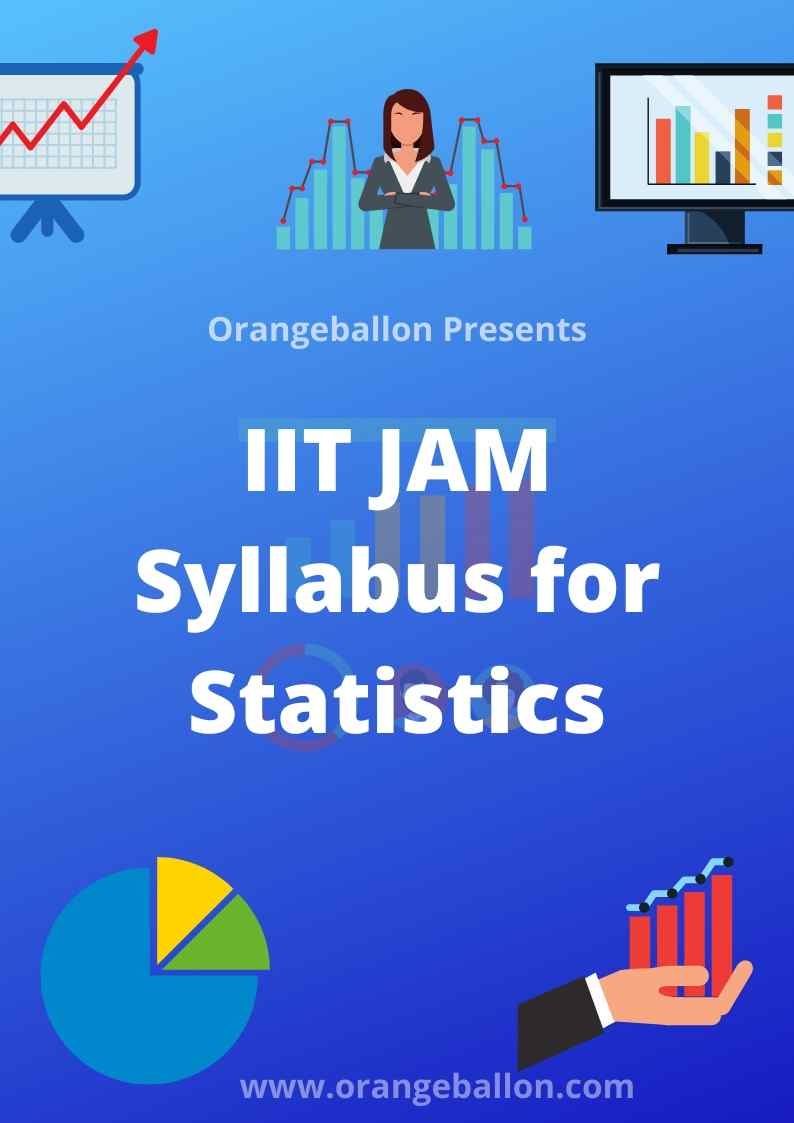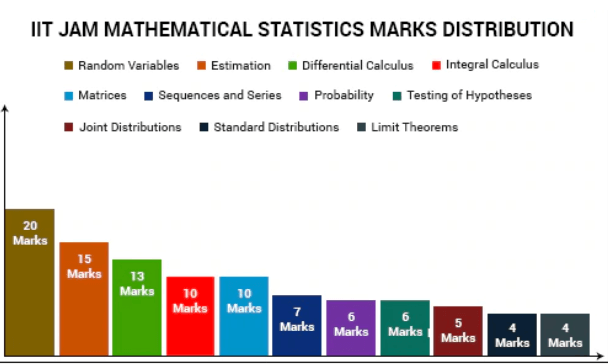```From the Academic Session 2004-05, IITs started conducting a Joint Admission Test for M.Sc. (JAM). There are many MSc entrance exams held across India and IIT JAM is the most popular among them.The objective of JAM is to provide admissions to M.Sc. (Two Years), Joint M.Sc.-Ph.D., M.Sc.-Ph.D. Dual Degree, and other Post-Bachelor Degree Programmes at the IITs and Integrated Ph.D. Degree Programmes at the IISc and to consolidate Science as a career option for bright students across the country. IIT JAM Examination is conducted in six subjects, also referred to as Test Papers; Biotechnology (BT), Chemistry (CY), Geology (GG), Mathematics (MA), Mathematical Statistics (MS) and Physics (PH).IIT JAM Mathematical statistics come with a bright future scope. Data scientist, data analyst, Quality analyst, Data Engineer, etc are some of the jobs offered to students from the statistical backgrounds.In this post, we will briefly give the syllabus of IIT JAM mathematical statistics.Content:1. IIT JAM Syllabus for Statistics2. The pattern of IIT JAM Statistics Test Papers3. IIT JAM Mathematical Statistics previous year papers4. Opening and Closing Ranks for IIT JAM 20205. IIT JAM Mathematical Statistics Seats from IITs6. Useful Tips for preparation of IIT JAM exam Mathematical StatisticsIIT JAM Syllabus for Statistics:The Mathematical Statistics (MS) test paper comprises of Mathematics (40% weightage) and Statistics (60% weightage). Mathematics Sequences and Series: Convergence of sequences of real numbers, Comparison, root and ratio tests for convergence of series of real numbers. Differential Calculus: Limits, continuity, and differentiability of functions of one and two variables. Rolle's theorem, mean value theorems, Taylor's theorem, indeterminate forms, maxima and minima of functions of one and two variables. Integral Calculus: Fundamental theorems of integral calculus. Double and triple integrals, applications of definite integrals, arc lengths, areas, and volumes. Matrices: Rank, the inverse of a matrix. Systems of linear equations. Linear transformations, eigenvalues, and eigenvectors. Cayley-Hamilton theorem, symmetric, skew-symmetric, and orthogonal matrices. Statistics Probability: Axiomatic definition of probability and properties, conditional probability, multiplication rule. The theorem of total probability. Bayes’ theorem and independence of events.

Random Variables: Probability mass function, probability density function, and cumulative distribution functions, distribution of a function of a random variable. Mathematical expectation, moments, and moment generating function. Chebyshev's inequality. Standard Distributions: Binomial, negative binomial, geometric, Poisson, hypergeometric, uniform, exponential, gamma, beta, and normal distributions. Poisson and normal approximations of a binomial distribution. Joint Distributions: Joint, marginal, and conditional distributions. Distribution of functions of random variables. Joint moment generating function. Product moments, correlation, simple linear regression. Independence of random variables. Sampling Distributions: Chi-square, t and F distributions, and their properties.Limit Theorems: Weak law of large numbers. Central limit theorem (i.i.d. with finite variance case only). Estimation: Unbiasedness, consistency, and efficiency of estimators, method of moments, and method of maximum likelihood. Sufficiency, factorization theorem. Completeness, Rao-Blackwell, and Lehmann-Scheffe theorems, uniformly minimum variance unbiased estimators. Rao-Cramer inequality. Confidence intervals for the parameters of univariate normal, two independent normals, and one parameter exponential distributions. Testing of Hypotheses: Basic concepts, applications of Neyman-Pearson Lemma for testing simple and composite hypotheses. Likelihood ratio tests for parameters of univariate normal distribution.Topic-wise Marks Distribution of IIT JAM Mathematics StatisticsThe pattern of IIT JAM Statistics Test Papers:The JAM 2020 Examination for all the six test papers will be carried out as ONLINE Computer Based Test (CBT) where the candidates will be shown the questions in a random sequence on a computer screen. For all the six test papers, the duration of the examination will be 3 hours. The medium for all the test papers will be English only.There will be a total of 60 questions carrying 100 marks. The entire paper will be divided into three sections, A, B, and C. All sections are compulsory. Questions in each section will be of different types as given below:Section–A contains a total of 30 Multiple Choice Questions (MCQs) involving 10 questions of one mark each and 20 questions of two marks each.Each MCQ has four choices out of which only one choice is the correct answer. Candidates can mark the answer by clicking the choice.Section–B contains a total of 10 Multiple Select Questions (MSQs) carrying two marks each. Each MSQ is similar to MCQ but with the difference that MSQ may have one or more than one correct choice(s) out of the four given choices. The candidate gets full credit only if he/she selects all the correct answer(s) only and no wrong answers. Candidates can mark the answer(s) by clicking the choice(s).